# Agar Cube Cell Size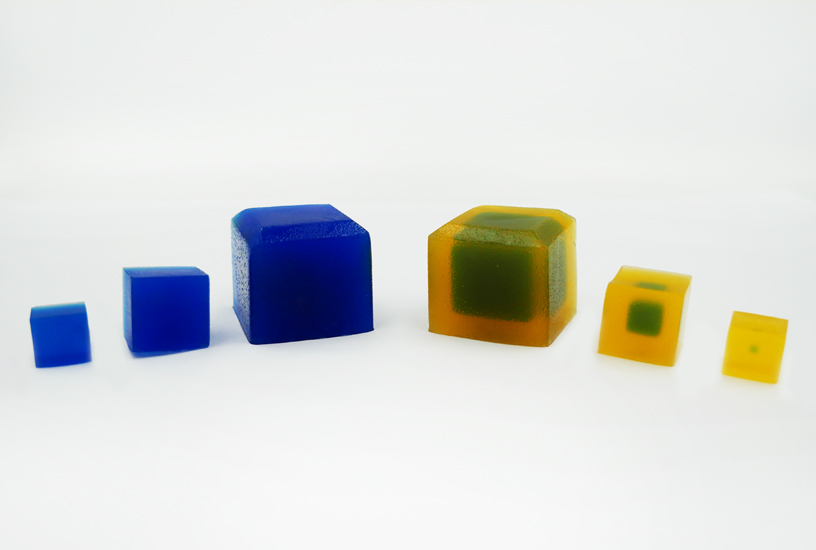### AUSTRALIAN CURRICULUM ALIGNMENT:

• Movement of materials across membranes occurs via diffusion, osmosis, active transport and/or endocytosis

### BACKGROUND:

The relatively small size of cells allows molecules in and out of their membranes. If a cell gets too large then the centre cannot be serviced efficiently. As the size of an object increases, the volume increases at a greater rate than surface area. For a cell, this means that efficiency of the exchange of materials across a membrane is reduced, and therefore the cell’s ability to take in enough nutrients is reduced. Furthermore, toxins may be retained for too long. Using Agar Cubes with indicator, vinegar and some simple mathematics, we can see how much effect a small increase in surface area has on volume.

In this practical, students are given Agar Cubes of three different sizes, impregnated with indicator to display an alkaline pH. Initial measurements make it clear that the surface-area-to-volume ratio is large for small cubes, with the ratio reducing as cube size increases. The cubes are placed in vinegar, and as the acid diffuses into the cell to reduce the pH and change the colour of the indicator, students see that a small difference in size can make a large difference to the time it takes to completely penetrate to the centre. Students also have the opportunity to calculate the results and plot graphs. Alternatively, a spreadsheet with the equations programmed in can be used.

### PREPARATION - BY LAB TECHNICIAN

• Remove Agar Cubes from packaging just prior to the experiment to avoid too much exposure to air.
• Place one large, medium and smaller sized cube on each workstation.

NOTE: It is best to leave the the cubes in the packaging in the fridge until ready for use.

### METHOD - STUDENT ACTIVITY

1. Measure each cube in height (h), width (w) and length (L) to calculate surface area (SA) and initial volume (Vi).
2. Fill the clear container with vinegar, enough that the largest cube can be submerged, and place one cube of each size into the container.
3. After five minutes, remove the cubes and measure the portion of each that is still blue. Try to minimise the amount of time the cubes are out of the vinegar.
4. Replace the cubes and then repeat leaving them in for five minutes at a time and measuring the dimensions of the blue portion after every five minutes until all the colour has gone.
5. Calculate the volume of the portion of the cube that is still blue (Vf) after each five-minute interval and the percentage of the whole cube that the vinegar has penetrated (%P).
6. Create a graph of time in minutes (X axis) against the %P (Y axis) of each cube. Comparing them all on one graph will demonstrate the differences in the trajectory of each.
7. Create a graph of initial surface-area-to-volume ratio (X axis) against the total time in minutes (Y axis) to demonstrate that as the ratio increases, the time taken to completely penetrate will decrease in a non-linear fashion.

### OBSERVATION AND RESULTS

Below is an example of the results table from this experiment. The calculations of surface area and volume show that the surface area of the largest cube is close to six times that of the smallest, while the volume has increased to about fourteen times that of the smallest. As well, the time taken for the cubes to lose colour has increased beyond what may be expected for the change in size.

Initial measurements:
SA = surface area
Vi = initial volume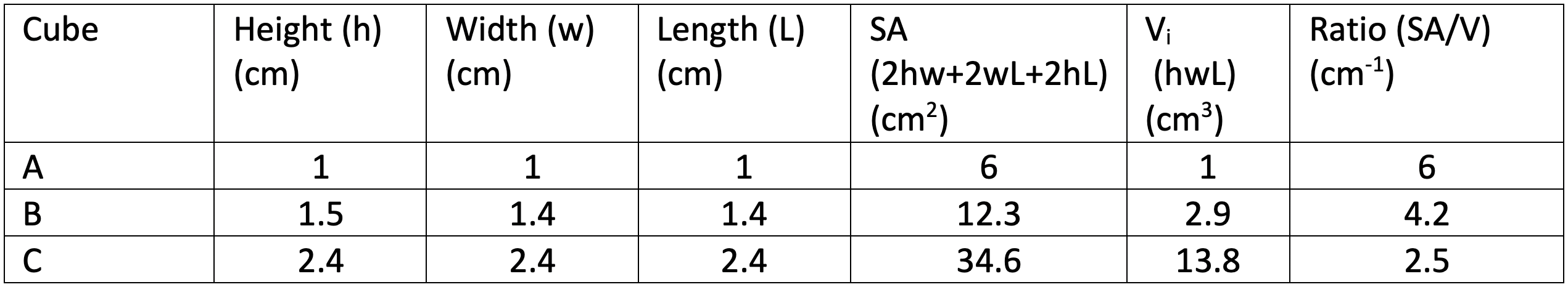Measurements over time:
P = penetration of the vinegar into the cube
Vi = initial volume (from table above)
Vf = volume of the part of the cube that is still blue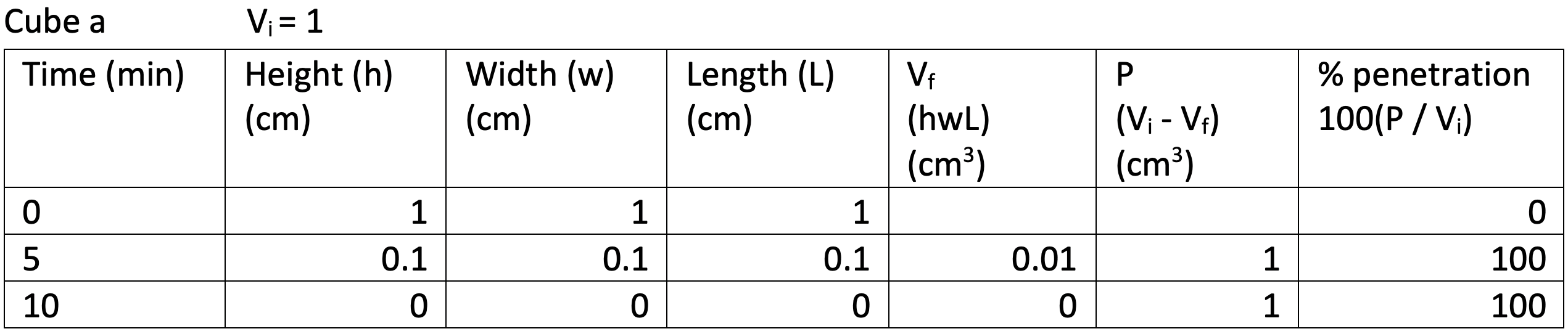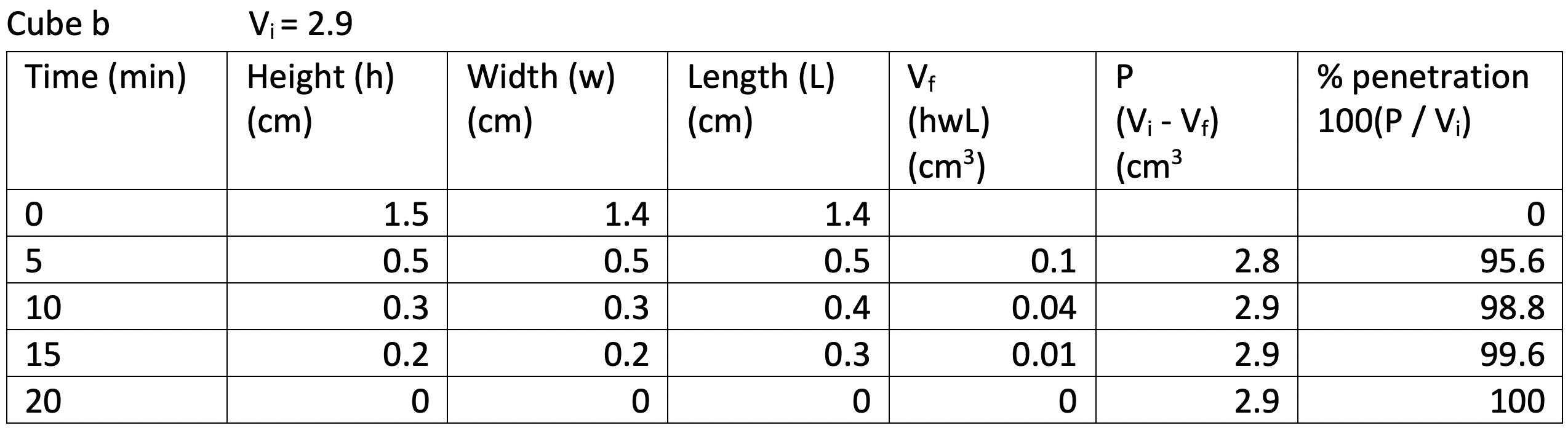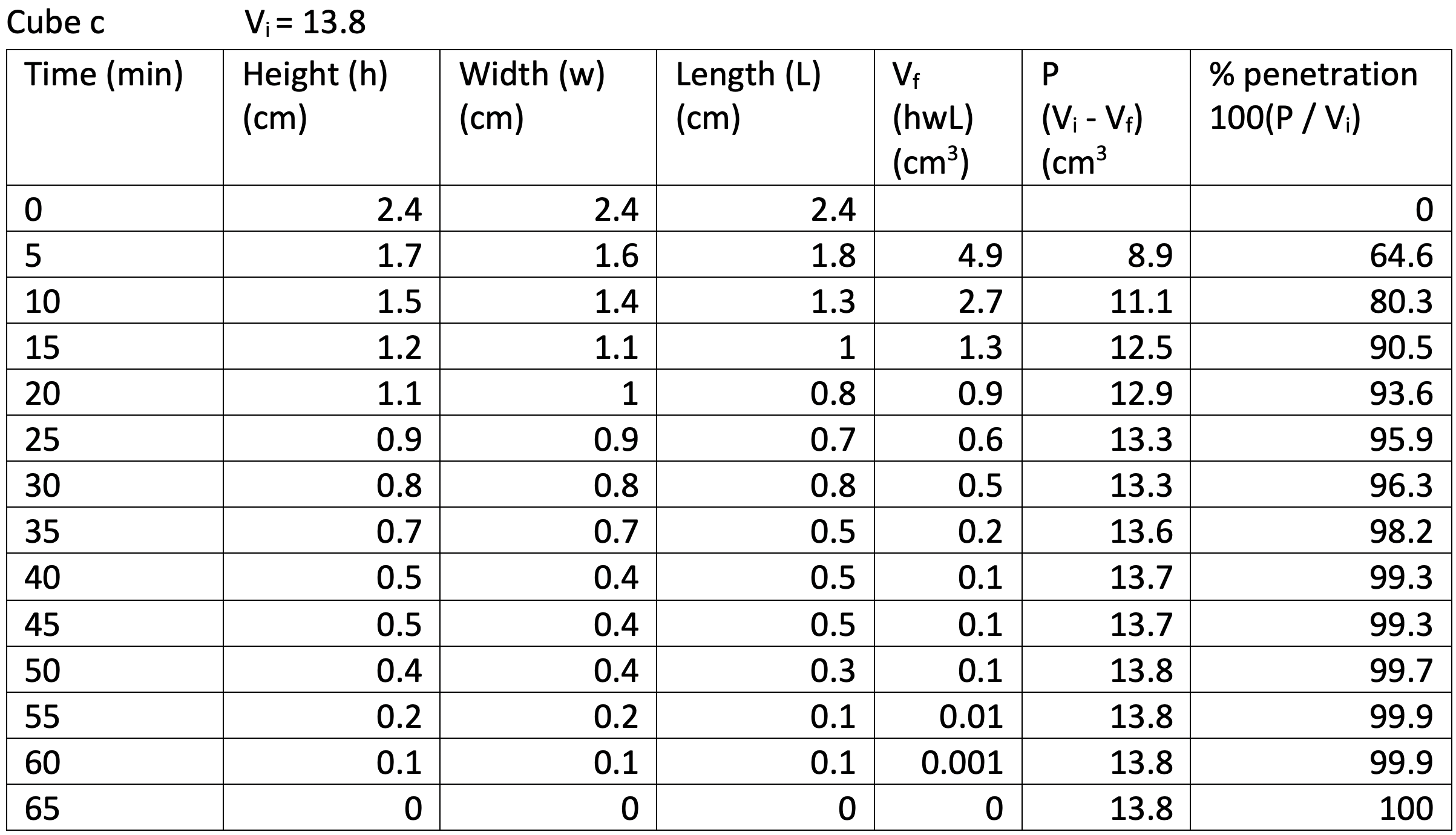###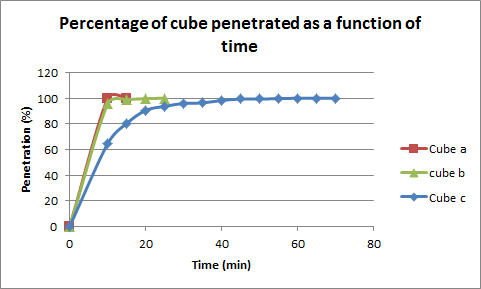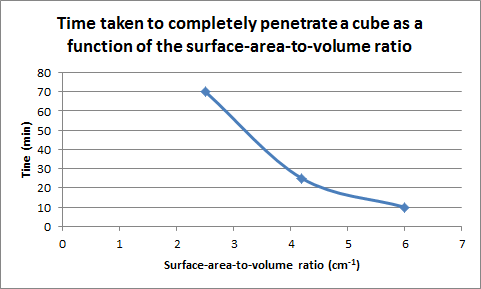### INVESTIGATION

Challenge your students to consider why the volume increases at a higher rate than the surface area as the dimensions of the cube increase. Ask your students to think about the way the numbers are calculated. Students may recognise that surface area is two numbers multiplied by each other, while volume is three numbers multiplied by each other. For example, 2x2=4 and 2x2x2=8, while 3x3=9 and 3x3x3=27. Although the surface area of one face is multiplied by six to get the total surface area of the cube, this number remains the same and does not increase as the cube increases. Note: multiplying by six works for a perfect cube. For an imperfect cube, the surface area of each face is calculated and then each is added together, but the concept remains the same.

The time it takes the vinegar to penetrate increases beyond what may be expected for the increase in dimensions; ask your students to consider possible reasons behind this. This may be helped by a demonstration with wooden blocks. A single cube will have all faces exposed, a cube of eight blocks has each one with at least one face exposed, and a cube of twenty-seven blocks will have one hidden in the centre.

### TEACHER TIPS:

• If Agar Cubes have turned green prior to the experiment, place them in a beaker in a 5% Bromothymol Blue solution and add Sodium Hydroxide using a dropping pipette until the cubes return to blue. Be aware this process may take a few hours.

1. 45 mins

#####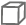Material List
1. Agar cube set
2. Vinegar
3. Clear container for immersing cubes, eg beakers or a bowl
4. Spoon
5. Timer
6. Ruler
7. Calipers (optional)
#####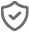Safety Requirements
1. Wear appropriate personal protective equipment (PPE)
2. Exercise caution when handling the vinegar as it may cause moderate skin and eye irritation.